# Section 11.4. Creating and Initializing an Array

[Page 356 (continued)]

### 11.4. Creating and Initializing an Array

Remember that we can declare an array using:

`type[] name;`

[Page 357]

But this doesn't actually create the array object! It only declares a variable that will refer to an array object. To create an array use:

`new type[numElements];`

or, if you want to initialize the elements of an array when you create it, you can use:

`type[] name = {elem1,elem2,elem3,...};`

So to create a Student object and pass in a name and an array of five grades you can use the following (see the result in Figure 11.9):

`> double[] gArray = {80, 90, 95, 87, 98}; > System.out.println(new Student("Ron Farmer", gArray)); Student object named: Ron Farmer`

##### Figure 11.9. Showing a student object after a constructor that takes a name and array of grades has executed.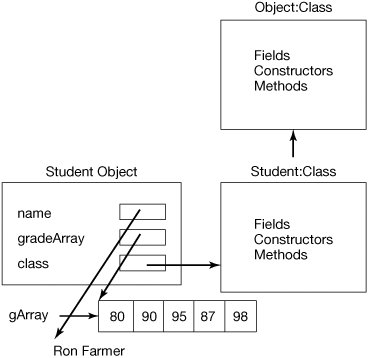Or you can create the array and separately fill in the grades:

`> double[] gArray = new double; > gArray = 80; > gArray = 90; > gArray = 95; > gArray = 87; > gArray = 98; [Page 358]> System.out.println(new Student("Ron Farmer", gArray)); Student object named: Ron Farmer`

It doesn't matter whether you initialize the array when you create it or after you create it using the indices. In this case the values in the array are exactly the same. If you initialize the array when you create it, the array length is set to the number of items between the curly braces.

#### 11.4.1. Calculating the Grade Average

It might be nice to change the toString method to add the average of the grades for this student to the string that it returns. Since this is something that objects of other classes might want to ask a Student object, we should make calculating the average a public method.

This method should first check if there is a grade array yet. If there isn't, it should return 0.0 for the grade point average. What if there are 0 grades? This could cause a divide by zero runtime exception. So we should also return 0.0 for the average if there are no grades in the array.

If there are grades in the grade array, we can sum the grades and divide by the number of grades to get the average. We can loop through the array, using a for loop that starts with an index of 0, and loops while the index is less than the length of the array. We will need to declare a variable to hold the sum. This should also be of type double since the grades are of type double. Each time through the loop we should add the current grade (at the current index value in the array of grades) to the sum. After the loop we can calculate the average as the sum divided by the number of grades, which is also the length of the array. Remember that you can get the length of an array using arrayObj.length.

##### Program 100. Student Class with Average Calculation (This item is displayed on pages 358 - 359 in the print version)`public class Student { //////////// fields ////////////////// private String name; private double[] gradeArray; //////////// constructors /////////// public Student() {} public Student(String theName) { this.name = theName; } public Student(String theName, double[] theGradeArray) { this.name = theName; [Page 359] this.gradeArray = theGradeArray; } /////////// methods /////////////// public double getAverage() { double average = 0.0; if (this.gradeArray != null && this.gradeArray.length > 0) { double sum = 0.0; for (int i = 0; i < this.gradeArray.length; i++) { sum = sum + this.gradeArray[i]; } average = sum / this.gradeArray.length; } return average; } public String toString() { return "Student object named: " + this.name + " Average: " + this.getAverage(); } }`

If we try this out we get:

`> double[] gArray = {80, 90, 95, 87, 98}; > System.out.println(new Student("Ron Farmer", gArray)); Student object named: Ron Farmer Average: 90.0 > double[] gArray2 = {92,94,97,91,93}; > System.out.println(new Student("Sue Lane",gArray2)); Student object named: Sue Lane Average: 93.4`

Since we made the method getAverage() public, we can also invoke it directly on a Student object from the interactions pane (which is outside the current class).

`> double[] gradeArray3 = {55, 85, 73, 92, 81}; > Student student1 = new Student("Bill Simpson",gradeArray3); > System.out.println(student1.getAverage()); 77.2`

#### 11.4.2. Using Step Into in the Debugger

We can use the debugger again to check that things are working the way that we expect. Click on DEBUGGER and check the DEBUG MODE checkbox. Create a breakpoint at the first line in the constructor that takes a name and a grade array (Figure 11.10). Create another breakpoint in the first line of the toString method. You can set as many breakpoints as you want.

[Page 360]

##### Figure 11.10. Breakpoint in the constructor that takes a name and grade array.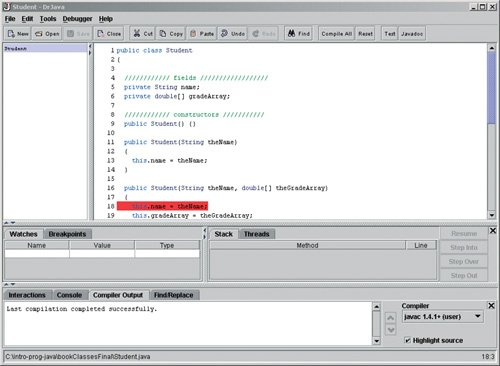Now let's execute this with

`> double[] gArray = {80, 90, 95, 87, 98}; > System.out.println(new Student("Ron Farmer", gArray));`

Execution will stop before the execution of the line that assigns the passed name to the current object's name (Figure 11.11). We can see that the current object fields have their default values by typing the following in the interactions pane. We can also check the values of the parameters to the constructor.

`> this.name null > this.gradeArray null > theName "Ron Farmer" > theGradeArray 80.0`

##### Figure 11.11. Execution stopped at the breakpoint. (This item is displayed on page 361 in the print version)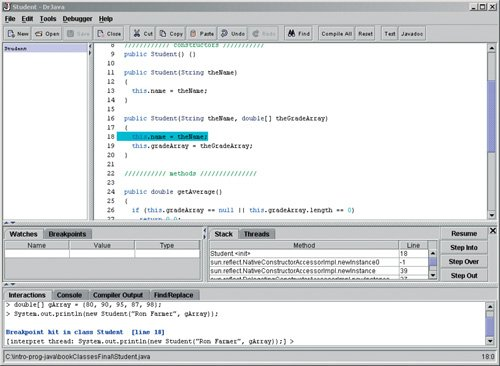We can use the STEP OVER button to execute the current line of code and then stop again after it has executed (Figure 11.12). If we do this, we can check the value of the current object's name again and see that it has been set to the value of the parameter.

[Page 361]

##### Figure 11.12. Execution stopped after step over.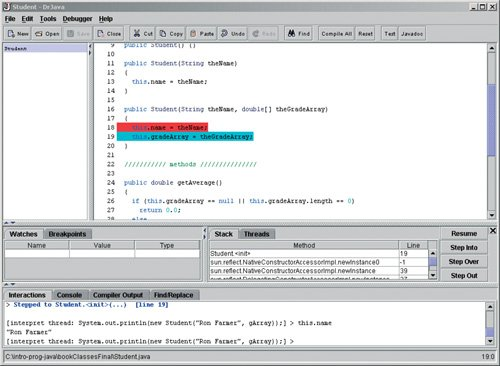[Page 362]

We can continue to click on Step Over to execute each line of code and stop again. We can use the interactions pane to print out the values that have been changed. Or at this point we can click on RESUME to let the program continue without stopping until the next breakpoint, or until it finishes.

Execution will stop at the second breakpoint in the method toString (Figure 11.13). We can see from the STACK tab that it is stopped at line 39 in the method toString in the class Student. If, at this point, we want to see what happens when we execute the this.getAverage() method we can use STEP INTO instead of STEP OVER. Using STEP INTO will take us into a method which is invoked on the current line. If we use STEP OVER it would just execute the method and stop after it. We wouldn't get to see what happens inside the method.

##### Figure 11.13. Execution stopped at the second breakpoint.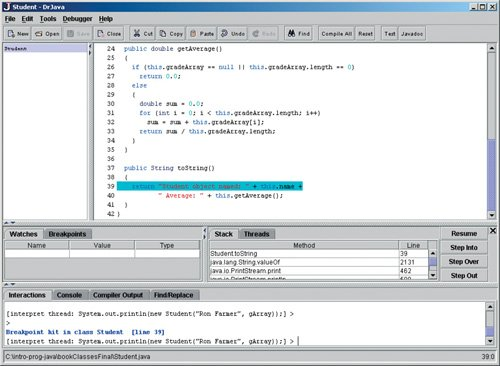After we use the STEP INTO button to step into the current line of code execution will stop at the first line of the getAverage method (Figure 11.14). We can use the STEP OVER button to execute each line in this method and stop after each to see what happened. We can check the value the of the sum variable each time through the loop. We can click RESUME when we want to continue execution.

##### Figure 11.14. Execution stopped at the beginning of getAverage. (This item is displayed on page 363 in the print version)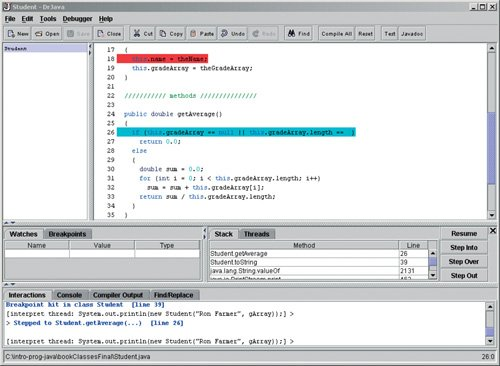If you ever use STEP INTO when you meant to use Step Over, all you have to do is click on STEP OUT. Clicking on STEP OUT will finish execution of the current method and stop at the line following the line that called the current method.Introduction to Computing & Programming Algebra in Java(c) A Multimedia Approach
ISBN: N/A
EAN: N/A
Year: 2007
Pages: 191